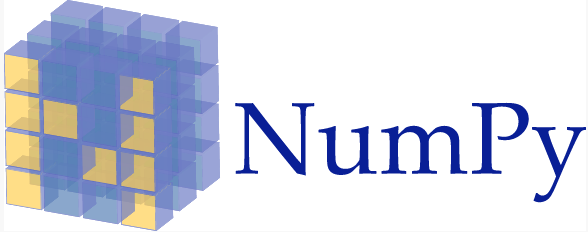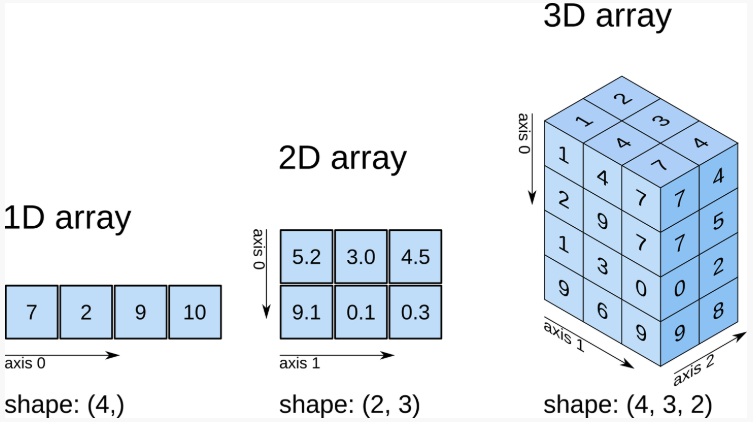# 小姐姐学Python-数据分析-Numpy数值计算基础1

## 前言

• 搬运，笔者也会从头学习一边
• 建议使用Jupyter notebook练习

#### 知识点

• 数值类型及多维数组
• 数组操作及随机抽样
• 数学函数及代数运算
• 数组索引及其他用法NumPy 的英文全称为 Numerical Python，意味 Python 面向数值计算的第三方库。NumPy 的特点在于，针对 Python 内建的数组类型做了扩充，支持更高维度的数组和矩阵运算，以及更丰富的数学函数。

NumPy 是 Scipy.org 中最重要的库之一，它同时也被 Pandas，Matplotlib 等我们熟知的第三方库作为核心计算库。当你在单独安装这些库时，你会发现同时会安装 NumPy 作为依赖。（后面我们会讲Pandas库，以及Matplotlib库）

#### NumPy 数组类型

• bool 布尔类型，1 个字节，值为 True 或 False。
• int 整数类型，通常为 int64 或 int32 。
• intc 与 C 里的 int 相同，通常为 int32 或 int64。
• intp 用于索引，通常为 int32 或 int64。
• int8 字节（从 -128 到 127）
• int16 整数（从 -32768 到 32767）
• int32 整数（从 -2147483648 到 2147483647）
• int64 整数（从 -9223372036854775808 到 9223372036854775807）
• uint8 无符号整数（从 0 到 255）
• uint16 无符号整数（从 0 到 65535）
• uint32 无符号整数（从 0 到 4294967295）
• uint64 无符号整数（从 0 到 18446744073709551615）
• float float64 的简写。
• float16 半精度浮点，5 位指数，10 位尾数
• float32 单精度浮点，8 位指数，23 位尾数
• float64 双精度浮点，11 位指数，52 位尾数
• complex complex128 的简写。
• complex64 复数，由两个 32 位浮点表示。
• complex128 复数，由两个 64 位浮点表示。

import numpy as np  # 导入 NumPy 模块

a = np.array([1.1, 2.2, 3.3])  # 指定 1 维数组的数值类型为 float64
a, a.dtype  # 查看 a 及 dtype 类型


a.astype(int).dtype


#### Numpy数组生成

• 列表：[1, 2, 3]
• 元组：(1, 2, 3, 4, 5)
• 字典：{A:1, B:2}

NumPy 中，ndarray 类具有六个参数，它们分别为：

• shape：数组的形状。
• dtype：数据类型。
• buffer：对象暴露缓冲区接口。
• offset：数组数据的偏移量。
• strides：数据步长。
• order：{‘C’，’F’}，以行或列为主排列顺序。

• 从 Python 数组结构列表，元组等转换。
• 使用 np.arange、np.ones、np.zeros 等 NumPy 原生方法。
• 从存储空间读取数组。
• 通过使用字符串或缓冲区从原始字节创建数组。
• 使用特殊函数，如 random。

#### 列表或元组转换

numpy.array(object, dtype=None, copy=True, order=None, subok=False, ndmin=0)


• object：列表、元组等。
• dtype：数据类型。如果未给出，则类型为被保存对象所需的最小类型。
• copy：布尔类型，默认 True，表示复制对象。
• order：顺序。
• subok：布尔类型，表示子类是否被传递。
• ndmin：生成的数组应具有的最小维数。

np.array([[1,2,3],[4,5,6]])


np.array([(1,2),(3,4),(5,6)])


#### arange方法创建

numpy.arange(start,stop,step, dtype=None)


# 在区间 [3, 7) 中以 0.5 为步长新建数组
np.arange(3, 7, 0.5, dtype='float32')


#### linspace 方法创建

linspace方法也可以像arange方法一样，创建数值有规律的数组。linspace 用于在指定的区间内返回间隔均匀的值。其方法如下：

linspace 方法创建
linspace方法也可以像arange方法一样，创建数值有规律的数组。linspace 用于在指定的区间内返回间隔均匀的值。其方法如下：

• start：序列的起始值。
• stop：序列的结束值。
• num：生成的样本数。默认值为50。
• endpoint：布尔值，如果为真，则最后一个样本包含在序列内。
• retstep：布尔值，如果为真，返回间距。
• dtype：数组的类型。
np.linspace(0, 10, 10, endpoint=True)


np.linspace(0, 10, 10, endpoint=False)


#### ones方法创建

numpy.ones 用于快速创建数值全部为 1 的多维数组。其方法如下：

numpy.ones(shape, dtype=None, order='C')


• shape：用于指定数组形状，例如（1， 2）或 3。
• dtype：数据类型。
• order：{‘C’，’F’}，按行或列方式储存数组。
np.ones((2, 3))


#### zeros 方法创建

zeros 方法和上面的 ones 方法非常相似，不同的地方在于，这里全部填充为 0。zeros 方法和 ones 是一致的。

np.zeros((3, 2))


#### eye 方法创建

numpy.eye 用于创建一个二维数组，其特点是k 对角线上的值为 1，其余值全部为0。方法如下：

numpy.eye(N, M=None, k=0, dtype=<type 'float'>)


• N：输出数组的行数。
• M：输出数组的列数。
• k：对角线索引：0（默认）是指主对角线，正值是指上对角线，负值是指下对角线。
# 可以通过改变第三个参数查看效果
np.eye(5,4,1)


#### 从已知数据创建

• frombuffer（buffer）：将缓冲区转换为 1 维数组。
• fromfile（file，dtype，count，sep）：从文本或二进制文件中构建多维数组。
• fromfunction（function，shape）：通过函数返回值来创建多维数组。
• fromiter（iterable，dtype，count）：从可迭代对象创建 1 维数组。
• fromstring（string，dtype，count，sep）：从字符串中创建 1 维数组。
# 结果没太搞明白？ 如果清楚结果的麻烦留言告诉我~
np.fromfunction(lambda a,b : a + b,(4,4))


#### ndarray 数组属性

a = np.array([[1, 2, 3], [4, 5, 6], [7, 8, 9]])
a  # 查看 a 的值


ndarray.T 用于数组的转置，与 .transpose() 相同。

a.T


ndarray.dtype 用来输出数组包含元素的数据类型。

a.dtype


ndarray.imag 用来输出数组包含元素的虚部。

a.imag


ndarray.real用来输出数组包含元素的实部。

a.real


ndarray.size用来输出数组中的总包含元素数。

a.size


ndarray.itemsize输出一个数组元素的字节数。

a.itemsize


ndarray.nbytes用来输出数组的元素总字节数。

a.nbytes


ndarray.ndim用来输出数组尺寸。

a.ndim


ndarray.shape用来输出数组维数组。

a.shape


ndarray.strides用来遍历数组时，输出每个维度中步进的字节数组。

a.strides


## 数组维度和形状

NumPy 数组又被称之为 ndarray 多维数组，那么 n 就可以从 1 维依次递增。下图，我们展示了 1 至 3 维的 NumPy 数组示例。1 维数组可以被看作数学中的向量，2 维数组可以看作是矩阵，而 3 维数组则是一个数据立方。

one = np.array([7,2,9,10])
two = np.array([[5.2, 3.0, 4.6],[9.1,0.1,0.3]])
three = np.array([[[ 1,1],[1,1],[1,1] ],
[[ 1,1],[1,1],[1,1] ],
[[ 1,1],[1,1],[1,1] ],
[[ 1,1],[1,1],[1,1] ]
])


one.shape, two.shape, three.shape


## 数组基本操作

#### 重设形状

reshape 可以在不改变数组数据的同时，改变数组的形状。其中，numpy.reshape() 等效于 ndarray.reshape()。reshape 方法非常简单：

numpy.reshape(a, newshape)


a = np.arange(10)
a = a.reshape((5,2))
a


np.arange(10).reshape((5,2))


#### 数组展开

ravel 的目的是将任意形状的数组扁平化，变为 1 维数组。ravel 方法如下：

numpy.ravel(a, order='C')


a = np.arange(10).reshape((2,5))
a

np.ravel(a)

np.ravel(a,order='F')


#### 轴移动

moveaxis 可以将数组的轴移动到新的位置。其方法如下：

numpy.moveaxis(a, source, destination)


• a：数组。
• source：要移动的轴的原始位置。
• destination：要移动的轴的目标位置。

a = np.ones((1,2,3))
a,a.shape

a = np.moveaxis(a,0,-1)
a,a.shape


#### 轴交换

numpy.swapaxes(a, axis1, axis2)


• a：数组。
• axis1：需要交换的轴 1 位置。
• axis2：需要与轴 1 交换位置的轴 1 位置。
a = np.ones((1,4,3))
a,a.shape

a = np.swapaxes(a,0,2)
a,a.shape


#### 数组转置

transpose 类似于矩阵的转置，它可以将 2 维数组的横轴和纵轴交换。其方法如下：

numpy.transpose(a, axes=None)


• a：数组。
• axis：该值默认为 none，表示转置。如果有值，那么则按照值替换轴。
a = np.arange(4).reshape(2, 2)
np.transpose(a)


#### 维度改变

atleast_xd 支持将输入数据直接视为 x维。这里的 x 可以表示：1，2，3。方法分别为：

numpy.atleast_1d()
numpy.atleast_2d()
numpy.atleast_3d()

print(np.atleast_1d([1, 2, 3]))
print(np.atleast_2d([4, 5, 6]))
print(np.atleast_3d([7, 8, 9]))
a = np.atleast_3d([7, 8, 9])
print(a.shape)
print(a,a,a)


#### 类型转换

* asarray(a，dtype，order)：将特定输入转换为数组。
* asanyarray(a，dtype，order)：将特定输入转换为 ndarray。
* asmatrix(data，dtype)：将特定输入转换为矩阵。
* asfarray(a，dtype)：将特定输入转换为 float 类型的数组。
* asarray_chkfinite(a，dtype，order)：将特定输入转换为数组，检查 NaN 或 infs。
* asscalar(a)：将大小为 1 的数组转换为标量。

a = np.arange(4).reshape(2, 2)
np.asmatrix(a)  # 将二维数组转化为矩阵类型


concatenate 可以将多个数组沿指定轴连接在一起。其方法为：

numpy.concatenate((a1, a2, ...), axis=0)


• (a1, a2, …)：需要连接的数组。
• axis：指定连接轴。
a = np.array([[1,2],[3,4],[5,6]])
b = np.array([[7,8],[9,10]])
c = np.array([[11,12]])

np.concatenate((a,b,c),axis=0)


a = np.array([[1, 2], [3, 4], [5, 6]])
b = np.array([[7, 8, 9]])

np.concatenate((a, b.T), axis=1)


## 参考

https://www.shiyanlou.com/courses/912/

1.图图说道：

np.fromfunction(lambda a,b : a + b,(4,4))
矩阵第i行第j列的数值为：i+j
因为定义了匿名函数a+b，创建数组时将i，j传入匿名函数，数组的结果等于函数的返回值

1.chaiwenjun000说道：

奈斯！谢谢！